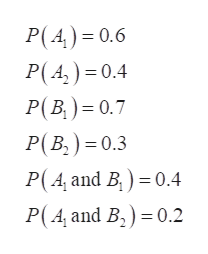# Using the below table:  A1A2B10.40.3B20.20.1 Compute P(A1 or B1).Compute P(A1 or B2).Calculate the marginal probabilities from the following table of joint probabilities.Determine P(A1 | B1).Determine P(A2 | B1).Did your answers to parts (a) and (b) sum to 1? Is this a coincidence? Explain.Calculate P(A1 | B2).Calculate P(A2 | B1).Are the events independent? Explain

Question
1. Using the below table:

 A1 A2 B1 0.4 0.3 B2 0.2 0.1

1. Compute P(A1 or B1).
2. Compute P(A1 or B2).
3. Calculate the marginal probabilities from the following table of joint probabilities.
4. Determine P(A1 | B1).
5. Determine P(A2 | B1).
6. Did your answers to parts (a) and (b) sum to 1? Is this a coincidence? Explain.
7. Calculate P(A1 | B2).
8. Calculate P(A2 | B1).

Are the events independent? Explain

check_circle

Step 1

Hello. Since your question has multiple sub-parts, we will solve first three sub-parts for you. If you want remaining sub-parts to be solved, then please resubmit the whole question and specify those sub-parts you want us to solve.

From the provided table the probabilities can be written as:help_outlineImage TranscriptioncloseP(A) 0.6 P(A)0.4 P(B) 0.7 P(B,) 0.3 P(A and B) 0.4 P(A and B,) 0.2 fullscreen
Step 2

1) The required probability of P(A1 or B1) can be calculated as:

Step 3

2)  The required probability of P(A1 or B2) can ...

### Want to see the full answer?

See Solution

#### Want to see this answer and more?

Solutions are written by subject experts who are available 24/7. Questions are typically answered within 1 hour.*

See Solution
*Response times may vary by subject and question.
Tagged in

### Other